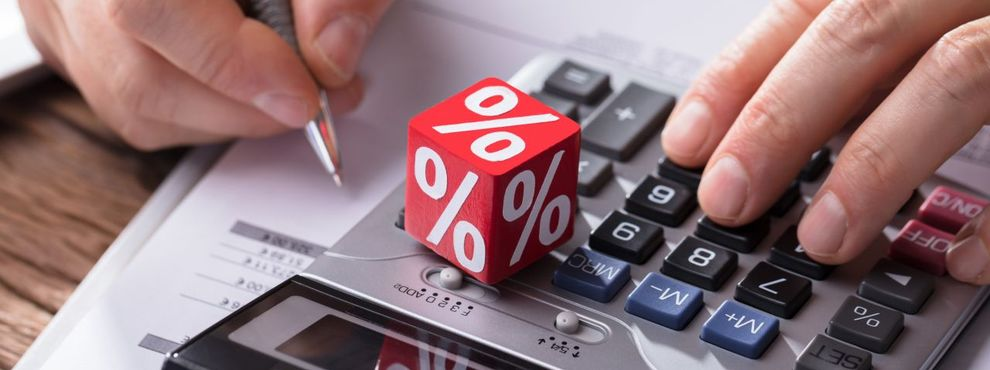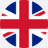# How to convert SGPA to percentage?Sruthi Ravinder
Last updated: Mar 28, 20237 min readGot a 6 CGPA and higher and planning to study abroad? Try our course finder.

## What is SGPA?

A figure called SGPA indicates a student's performance for a semester. The student's semester grade point average (SGPA) is the weighted average of all grade points earned in all courses taken during the semester. Nowadays, most institutes provide an SGPA to percentage converter or a calculator to cumulate the points into a percentage equivalent. This data will be the one considered by the admissions committee of various colleges to take in and assess your application.

So, let’s have a look at how this plays a key role in enhancing your higher education applications!

Synopsis

## How to Calculate SGPA?

• Write down all your credit points for each subject.
• Next, multiply the credit point of each topic with the grade that you achieved in that subject.
• Add all the scores that come.
• Next, divide the total score you got by the sum of all the credit points.
• The number that you get is the SGPA.

## How to convert SGPA into percentage?

• First, calculate the SGPA of all the semesters.
• Once you get the SGPA of all the semesters, add them.
• Divide the summation of the SGPAs by the number of semesters you have given.
• Multiply your SGPA with 10 and then subtract 7.5 from the total [(SGPA * 10) – 7.5 = Percentage].

## Convert SGPA to percentage

To convert SGPA to Percentage, we need to multiply SGPA by 10 and then subtract it with the 7.5 from the result, which will give us the percentage.

## SGPA to Percentage Calculator

Got a 6 CGPA and higher and planning to study abroad? Try our course finder.

## Difference between CGPA and SGPA

CGPA means Cumulative Grade Point Average and is the measuring grade for your overall performance during an academic year. SGPA is Semester Grade Point Average that adds all the CGPAs after an educational program.

Related articles

## How to calculate SGPA from marks?

Write down all your credit points for each subject.

• Add all the scores that come.
• Next, divide the total score you got by the sum of all the credit points.
• The number that you get is the SGPA.

## How to calculate SGPA in engineering?

Engineering examination results are denoted as SGPA & CGPA. SGPA & CGPA are calculated based on credits & grades. Then the SGPA to percentage converter will give you the resultant percentage equivalent.

## SGPA calculator for different Indian Universities

### How to convert SGPA into a percentage in Pune university?

According to them, to convert your SGPA/CGPA to a percentage, you need to multiply your grade by 8.8.

### How to convert SGPA into a percentage in AKTU university?

The SGPA is the ratio of the sum of the product of the number of credits to grade points scored by a student in all the courses taken by a student and the sum of the number of credits of all the courses undergone by a student. So,

where Ci is the number of credits of the nth course.

### How to calculate SGPA at JNTUH university?

A student earns a grade point (GP) in each subject/ course based on the letter grade secured in that subject/ course. The corresponding 'credit points' (CP) are computed by multiplying the grade point with credits for that particular subject/ course.

### How to calculate SGPA in VTU?

The VTU adopts an absolute grading system wherein the marks are converted to grades, and every semester's results will be declared with semester grade point average (SGPA) and Cumulative Grade Point Average (CGPA). They will calculate the CGPA every semester, except the first semester.

Related pages,

### How to convert SGPA to Percentage?

Multiply (*) the SGPA with ten and then subtract 7.5 from the results to get the percentage. This will give you the percentage.

### SGPA to CGPA, then to percentage conversion table

Participate in Edvoy’s refer & earn. Refer a friend to study abroad with Edvoy and be rewarded with vouchers, scholarships.

Book for a Remote Counselling to get started!

0 LikesWritten By
Sruthi Ravinder

A fun-loving journalist with a passion to get the information out there!